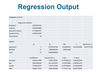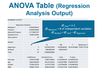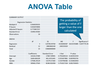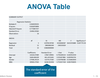# MID TERM REVIEW Flashcards Preview

## 1P97 ITIS - Data Anaylsis & Modelling > MID TERM REVIEW > Flashcards

Flashcards in MID TERM REVIEW Deck (69):
1

2

3

4

5

6

7

8

9

10

11

12

13

14

15

16

17

18

19

20

21

22

23

24

25

26

27

28

29

30

31

32

33

34

35

36

## In the ANOVA table what is the meaning of the regression stats? Ie. R, R^2 etc...

### C - Multiple R = coefficient of correlation - the measure of strength of the relationship between variables.  = the square root of R^2D - R^2 = Coefficient of determination - The percent of the variability in the dependent variable (Y) that is explained by the regression equation. Higher the better. Calculated by dividing SSR by SSTAdjusted R Square - A measure of the explanatory power of a regression model that takes into consideration the number of independent variables in the model.37

## In the ANOVA table. Explain the df column.

### Df - degree of freedomRegression DF = number of independent variablesResidual DF = total df - df regressionTotal DF = total observations - 1 (n-1)38

## In the ANOVA table, explain the SS column.

### SSR (on top), SSE(middle), and SSTotal (SSR and SSE sum) are results from the regression analysis, they inform other columnsSSR / SST = R^239

## In the ANOVA table, Explain the MS column

### MSR Regression = SSR / DF regressionMSE Residual = SSE / DF residual40

## In the ANOVA table, how if F calculated? What is it?

### F = MSR / MSEThis is the test statistic. It informs Signifgance F41

## In the ANOVA table, What is Signifgance F? What does it mean?

### It is the probability of getting a value of F larger than the one calculated. The lower the Sig F the better and more statistically significant the model is.Ex. It should be less than your stated level of signifgance (.05, .01...95%, 99% etc)42

## In the ANOVA table, explain the Coefficients column

### Intercept is the a value of the y=a + bxThe lines that follow are the b variables values43

## What is the Standard Error column in the ANOVA table?

### The standard deviation of the errors, sometimes called the standard deviation of the regression.Error is the difference between the actual Y value and the predicted value. Residual is another term for error.44

## In the ANOVA table what is the P-value column?

### This is, by variable, the probability of getting a t stat larger than the one calculated. Lower is better here. A high value may lead to rejection of a variable (in concert the variable effect on the Adjusted R square value)45

46

47

48

49

50

51

52

53

54

55

56

57

58

59

60

61

62

63

64

65

66

67

68

69